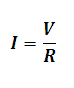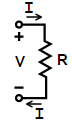# Ohm's Law Calculator

This Calctown Calculator calculates the electric current given the voltage and resistance.

V
ohm

#### Result

AClick here to view image

where

I = electric current

V = Voltage or potential difference

R = Resistance

eg. For V = 2V, R = 0.5ohm; I = V/R = 2/0.5 = 4A.